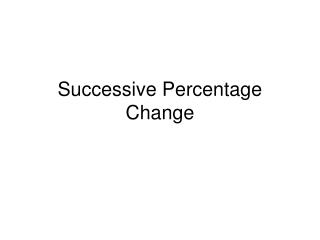DownloadDownload PresentationSuccessive Percentage Change

# Successive Percentage Change

Télécharger la présentation## Successive Percentage Change

- - - - - - - - - - - - - - - - - - - - - - - - - - - E N D - - - - - - - - - - - - - - - - - - - - - - - - - - -
##### Presentation Transcript

1. Successive Percentage Change

2. Jeans are on sale for 40% off the retail price. The retail price is \$40.00. If you have a coupon, you can receive an additional 20% off the sale price. What is the overall percentage savings? Determine the sale price: \$40 – (\$40 * .40) = \$24 Determine the final price with coupon: \$24 – (\$24 * .20) = \$19.20 Determine the overall percentage change: new – old = \$19.20 - \$40 = - 0.52 old \$40 -0.52 * 100% = Savings of 52%

3. VISUALLY Determine the sale price: \$40 – (\$40 * .40) = \$24 Determine the final price with coupon: \$24 – (\$24 * .20) = \$19.20 Determine the overall percentage change: new – old = \$19.20 - \$40 = - 0.52 old \$40 -0.52 * 100% = Savings of 52%

4. The Formula (1 ± P1) * (1 ± P2) – 1 = % where % is written in fractional form (decimal) P1 = First percentage increase/decrease P2 = Second percentage increase/decrease

5. Deriving the Formula • The overall percentage change does NOT depend on the Beginning Value (B). Can we show this by determining a process (formula) that includes just the two percents?

6. Deriving the Formula (cont.) • Variables B = Beginning Value I = Intermediate Value F = Final Value P1 = First Percent Decrease P2 = Second Percent Decrease

7. Deriving the Formula (cont.) First find the intermediate price B – (B * P1) = I Then find the final price I – (I * P2) = F Then find the overall percentage change F - B B

8. Deriving the Formula We can rewrite the first equation: B – (B * P1) = I As B * ( 1 – P1) = I We can rewrite the second equation: I – (I * P2) = F As I * (1 – P2) = F

9. Deriving the Formula (cont.) Substitute these into final equation: F – B B So , since we found that F = I*(1-P2) I*(1-P2) – B B And we also know that I = B*(1-P1) B*(1-P1) * (1-P2) – B B The B’s cancel out to arrive at: (1-P1) * (1-P2) - 1